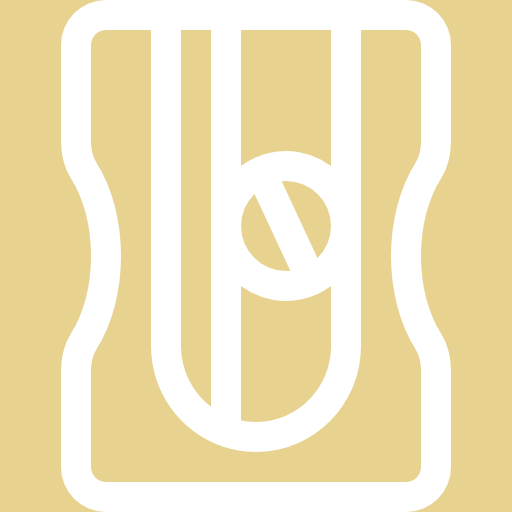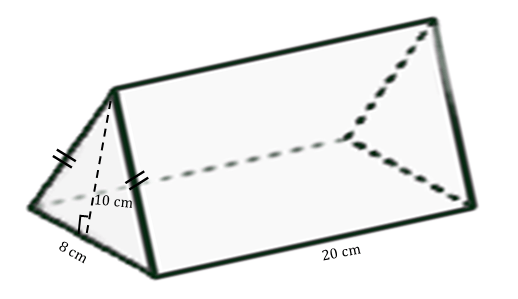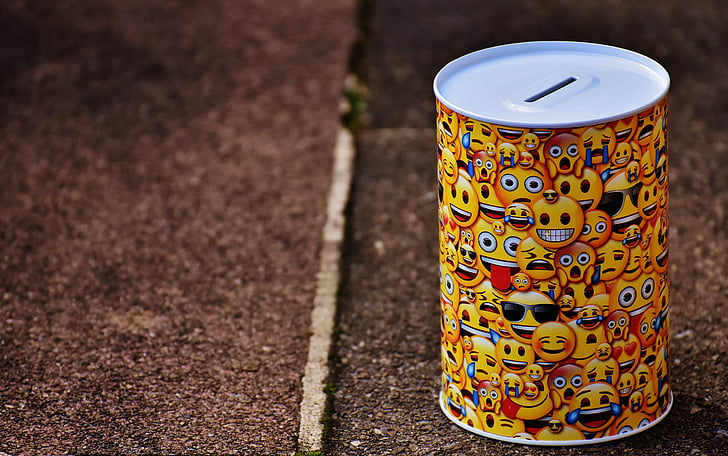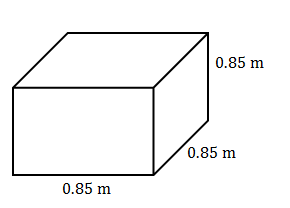Space, shape and measurement: Calculate perimeter, surface area and volume in two- and three-dimensional geometrical shapes

# Unit 2: Volume of 3-dimensional shapes

Natashia Bearam-Edmunds### Unit outcomes: Unit 2: Volume of 3-dimensional shapes

By the end of this unit you will be able to:

• Calculate the volume of the following geometric objects:
• Cubes
• Rectangular prisms
• Triangular prisms
• Hexagonal prisms
• Cylinders.
• Investigate the effect on the volume of right prisms and cylinders, where one or more dimensions are multiplied by a constant factor k.

## What you should know

Before you start this unit, make sure you can:

## Introduction

A 3-dimensional (3-D) shape is a solid or an object with three dimensions; length, width (also called breadth) and height. We saw in Unit 1 that polygons make up the bases and sides, also called faces, of 3-D objects. Every 3-D solid without a curved surface has a face, edge and vertex.

A face is a flat surface of a 3-D object. An edge is a line segment where two faces meet. A vertex is the point where the edges meet. A shape that has these properties is called a polyhedron. Examples of polyhedrons are the square pyramid (see Figure 2) and the dodecahedron (see Figure 3).

## Prisms

There are different types of polyhedrons but in this unit we will only focus on one type called prisms. Prisms have the same shape (face) on the top and on the base and these faces are parallel to each other. A parallelepiped (see Figure 4) is an example of a prism. Here the base and top are both rectangles.

A cube (see Figure 5) is also an example of a prism. It has square faces on the base and on the top (and on its sides too). But, a cube is a special kind of prism called a right prism. In a right prism the side faces are perpendicular or at right-angles to the base. In a right prism, the sides, or faces are always rectangles. Remember that all squares are also rectangles.

Solids with curved surfaces, such as cylinders, spheres and cones are not polyhedrons.

You come across 3-D objects every day. A toilet paper roll is cylindrical in shape. Dice are cubic. A beach ball is spherical and a party hat is cone shaped. Can you name a few other everyday items that are shaped like cylinders, cubes, spheres or cones?

## Volume of prisms

So far we have seen that in prisms the top and the base are the same polygon and are parallel to each other. In right prisms the side faces are perpendicular to the base and top and are rectangles.

There is another type of prism called an oblique (slanting) prism. These are like the parallelepiped in Figure 4. In oblique prisms the sides, or faces, are not perpendicular to the base and top. We will only cover finding the volume of right prisms and cylinders in this unit and for exam purposes.### Activity 2.1: Identify prisms

Time required: 10 minutes

What you need:

• a pen and paper

What to do

Study the right prisms below and answer the questions that follow.1. What are the top and base polygons in each case?
2. How many faces does each object have?
3. How many edges does each object have?
4. How many vertices does each object have?
5. Based on your answer to question 1, and if we name prisms according to the base faces, what do think each prism is called?

What did you find?

The answers are in the table below.

 Top and base polygons Number of faces Number of edges Number of vertices Prism name a) Triangles 5 faces 9 edges 6 vertices Triangular base so triangular prism b) Squares 6 faces 12 edges 8 vertices Square-based prism, cuboid or rectangular prism c) Hexagons 8 faces 18 edges 12 vertices Hexagonal prism d) Pentagons 7 faces 15 edges 10 vertices Pentagonal prism

### Note

For more on prisms and other 3-D shapes watch the video called “Three dimensional shapes” when you have access to the internet.

Three dimensional shapes (Duration: 08.18)Table 1 shows a summary of the common prisms and 3-D solids you will come across in this unit and their properties.

Recognising different types of 3-D objects so we can find the volume and surface area has important applications in many industries. For example, in manufacturing knowing the volume and surface area is necessary to minimise costs and maximise production.

Volume is the amount of 3-D space taken up by a solid, liquid or gas. Volume is measured in cubes or cubic units.

If you look at the label of any cold drink can, you will see the volume of liquid inside the container. Manufacturers pay close attention to the volume of their products to make sure they deliver an accurate amount every time. You would not be happy if a bottle was marked as containing $\scriptsize 400\text{ ml}$ of a product but only contained $\scriptsize 300\text{ ml}$ when you open it!

Can you tell just by looking at the objects below which one takes up more space?

Count the number of cubes that make up each object. There are $\scriptsize 4$ cubes that make up the first object and $\scriptsize 4$ cubes that make up the second object. Both objects occupy the same amount of space because their volumes are both $\scriptsize 4$ cubic units. So by counting how many cubes it will take to fill up an object we can calculate its volume.

Count the cubes that make up the solids shown in Figure 8. What is the volume of each one? Which object takes up more space?

There are $\scriptsize 8$ cubes that make up Figure 8A so its volume is $\scriptsize 8$ cubic units. There are $\scriptsize 12$ cubes that make up Figure 8B so its volume is $\scriptsize 12$ cubic units. Therefore, Figure 8B takes up more space as it has the bigger volume.

Breaking 3-D objects into cubes and counting them is a long and inefficient way to find the volume of an object. Counting in this way can also lead to careless mistakes. There is a much easier way to calculate the volume using a simple formula, which we discuss in the example that follows.

The volume of a right prism is calculated by multiplying the area of the base by the height of the solid.

$\scriptsize \text{V}=\text{Area of base}\times \text{height}$### Example 2.1

1. An ice cube has a side of $\scriptsize 2\text{ cm}$. What is the volume of the ice cube?
2. A swimming pool is shaped as a rectangular prism. It is $\scriptsize 6\text{ m}$ long, $\scriptsize \text{3 m}$ wide and $\scriptsize \text{2 m}$ deep. How much water is needed to completely fill the swimming pool?
3. A cylinder-shaped glass has a radius of $\scriptsize \text{4 cm}$ and height of $\scriptsize \text{6 cm}$. If the glass is half filled with water what is the volume of water in the glass?

Solutions

1. All the sides and height of a cube have the same measurement.
$\scriptsize \begin{array}{l}{{\text{V}}_{{\text{cube}}}}&=\text{area }\!\!~\!\!\text{ square }\!\!~\!\!\text{ base }\!\!~\!\!\text{ }\times \text{height }\!\!~\!\!\text{ of }\!\!~\!\!\text{ cube}\\&=\text{(}2\text{ cm}\times 2\text{ cm)}\times 2\text{ cm}\\&=8\text{ c}{{\text{m}}^{3}}\end{array}$
2. We use the formula $\scriptsize \text{V}=\text{Area of base}\times \text{height}$.
In this case the base is a rectangle.
$\scriptsize \begin{array}{l}\text{V}&=(l\times b)\times h\\&=(6\text{ m}\times 3\text{ m})\times 2\text{ m}\\&=36\text{ }{{\text{m}}^{3}}\end{array}$
3. Here we need to remember the formula for the area of a circle because a cylinder has a circular base.
First, calculate the total volume of the glass.
$\scriptsize \text{V}=\text{Area of base}\times \text{height}$
$\scriptsize \begin{array}{l}{{\text{V}}_{{\text{glass}}}}&=\text{Area of circle}\times \text{height}\\&=\pi {{r}^{2}}\times \text{h}\\&=\pi {{(4\text{ cm})}^{2}}\times 6\text{ cm}\\&=301.6\text{ c}{{\text{m}}^{3}}\end{array}$
Next, find a half the volume of the glass to calculate the actual volume of water in the glass.
$\scriptsize \begin{array}{l}{{\text{V}}_{{\text{water}}}}&=301.6\text{ c}{{\text{m}}^{3}}\times \displaystyle \frac{1}{2}\\&=150.8\text{ c}{{\text{m}}^{3}}\end{array}$### Exercise 2.1

1. Find the volume of this triangular prism.2. Find the volume of a cylinder with a radius of $\scriptsize \text{40 cm}$ and a height of $\scriptsize \text{1}\text{.5 m}$ (correct to 1 decimal place).
3. A cylindrical jug has a diameter of $\scriptsize \text{142 mm}$ and a height of $\scriptsize \text{28 cm}$. Will the jug be able to hold $\scriptsize 5\text{ l}$ of water? (HINT $\scriptsize \text{1 000 c}{{\text{m}}^{\text{3}}}\text{=1 l}$).

The full solutions are at the end of the unit.

## Multiplying dimensions by a factor of k

When one or more of the dimensions of a prism or cylinder is multiplied by a constant, the volume will change.

Once we understand the relationship between the change in dimensions and the resulting change in volume it becomes simpler to calculate the new volume of an object when its dimensions are scaled up or down.### Example 2.2

Consider a rectangular prism with dimensions $\scriptsize \text{l, b}$ and $\scriptsize \text{h}$. Complete the table to see how multiplying the dimensions by a scale factor affects the volume.

 Dimensions Volume Original dimensions $\scriptsize \text{l, b}$ and $\scriptsize \text{h}$ Multiply one dimension by $\scriptsize 3$ $\scriptsize \text{l, b}$ and $\scriptsize 3\text{h}$ Multiply two dimensions by $\scriptsize 3$ $\scriptsize \text{l, 3b}$ and $\scriptsize 3\text{h}$ Multiply all three dimensions by $\scriptsize 3$ $\scriptsize 3\text{l, 3b}$ and $\scriptsize 3\text{h}$ Multiply all three dimensions by $\scriptsize k$ $\scriptsize k\text{l, }k\text{b}$ and $\scriptsize k\text{h}$

Solutions

 Dimensions Volume Original dimensions $\scriptsize \text{l, b}$ and $\scriptsize \text{h}$ $\scriptsize \begin{array}{l}\text{V}=\text{l}\times \text{b}\times \text{h}\\=\text{lbh}\end{array}$ Multiply one dimension by $\scriptsize 3$ $\scriptsize \text{l, b}$ and $\scriptsize 3\text{h}$ $\scriptsize \begin{array}{l}{{\text{V}}_{1}}=\text{l}\times \text{b}\times 3\text{h}\\=3\text{lbh}\\=3\text{V}\end{array}$ Multiply two dimensions by $\scriptsize 3$ $\scriptsize \text{l, 3b}$ and $\scriptsize 3\text{h}$ $\scriptsize \begin{array}{l}{{\text{V}}_{2}}=\text{l}\times 3\text{b}\times 3\text{h}\\=3\times 3\text{lbh}\\={{3}^{2}}\text{V}\end{array}$ Multiply all three dimensions by $\scriptsize 3$ $\scriptsize 3\text{l, 3b}$ and $\scriptsize 3\text{h}$ $\scriptsize \begin{array}{l}{{\text{V}}_{3}}=3\text{l}\times 3\text{b}\times 3\text{h}\\=3\times 3\times 3\text{lbh}\\={{3}^{3}}\text{V}\end{array}$ Multiply all three dimensions by $\scriptsize k$ $\scriptsize k\text{l, }k\text{b}$ and $\scriptsize k\text{h}$ $\scriptsize \begin{array}{l}{{\text{V}}_{1}}=k\text{l}\times k\text{b}\times k\text{h}\\=k\times k\times k\text{lbh}\\={{k}^{3}}\text{V}\end{array}$

### Note

• If one dimension of a prism is multiplied by a factor of $\scriptsize k$ the volume of the prism is changed by a factor of $\scriptsize k$.
• If two dimensions of a prism are multiplied by a factor of $\scriptsize k$ the volume of the prism is changed by a factor of $\scriptsize {{k}^{2}}$.
• If all three dimensions of a prism are multiplied by a factor of $\scriptsize k$ the volume of the prism is changed by a factor of $\scriptsize {{k}^{3}}$.

Using the above information we can easily calculate the new volume of a prism. If, for example, the height is doubled, the new volume will also be twice the original volume.### Exercise 2.2

1. Consider a rectangular prism with a height of $\scriptsize \text{4 cm}$ and base lengths of $\scriptsize \text{3 cm}$.
1. Calculate the volume.
2. Calculate the new volume if the base lengths are multiplied by a constant factor of $\scriptsize \text{4}$.
3. Express the new volume as a factor of the original volume.
2. If the volume of a cylinder is $\scriptsize \text{V}=8\pi$, what will the new volume be if the radius is multiplied by $\scriptsize 2$?
3. Given a prism with a volume of $\scriptsize 493\text{ c}{{\text{m}}^{3}}$, find the new volume for a prism if all dimensions are multiplied by a constant factor of $\scriptsize 5$.

The full solutions are at the end of the unit.

## Summary

• In this unit you have learnt the following:
• How to identify different types of prisms based on their properties.
• How to find the volume of right prisms.
• How to find the volume of cylinders.
• How the volume is affected when the dimensions are multiplied by a constant factor.

# Unit 2: Assessment

#### Suggested time to complete: 20 minutes

1. Alfred uses a ‘piggy bank’ that is cylindrical in shape. The ‘piggy bank’ is constructed from tin and has a slot at the top. The area of the slot is $\scriptsize 2\text{ c}{{\text{m}}^{2}}$. The diameter of the base of the ‘piggy bank’ is $\scriptsize 11\text{ cm}$ and the height of the ‘piggy bank’ is $\scriptsize 7\text{ cm}$.Determine the volume of the ‘piggy bank’.
2. Thabo has glued together five square pieces of glass to build a fish tank which is open at the top. The length, breadth and height of the tank are all $\scriptsize 0.85\text{ m}$. The completed fish tank is shown below.1. Thabo wants to test his new tank for water leaks. Determine the volume of the water, in $\scriptsize {{\text{m}}^{3}}$, he would need to fill the tank to the top. (Ignore the thickness of the glass in your calculations).
2. Thabo wants to buy a glass lid for his tank. To prevent the lid from falling into the tank, he decides to buy a lid which is $\scriptsize 0.01$ times longer than the original length of each side of the tank. Determine the area (in $\scriptsize {{\text{m}}^{2}}$) of the glass Thabo needs to buy for the lid.

The full solutions are at the end of the unit.

# Unit 2: Solutions

### Exercise 2.1

1. ..
$\scriptsize \begin{array}{l}\text{Volume}&=\text{Area of base}\times \text{height}\\&\text{=Area of triangle}\times \text{height}\\&=(\displaystyle \frac{1}{2}\text{bh})\times 20\text{ }\\&\text{=(}\displaystyle \frac{1}{2}\cdot 8\cdot 10)\times 20\\&=800\text{ c}{{\text{m}}^{2}}\end{array}$
2. Make sure that the radius and height are in the same units before finding the volume. Convert either the radius to metres or the height to centimetres.
.
$\scriptsize \begin{array}{l}\text{40 cm}&=\displaystyle \frac{{40}}{{100}}\text{ m (}1\text{ cm}&=0.01\text{ m)}\\&=0.4\text{ m}\end{array}$
$\scriptsize \begin{array}{l}\text{V}&=\text{Area of base}\times \text{height}\\&\text{=}\pi {{\text{r}}^{2}}\times \text{h}\\&\text{=}\pi {{\text{(0}\text{.4)}}^{2}}\times 1.5\\&=0.8\text{ }{{\text{m}}^{3}}\text{ Or }800\text{ }000\text{ c}{{\text{m}}^{3}}\text{ (1 }{{\text{m}}^{3}}&=1\text{ }000\text{ }000\text{ c}{{\text{m}}^{3}})\end{array}$
3. First calculate the volume of the jug, remembering to change the diameter from$\scriptsize \text{mm}$ to $\scriptsize \text{cm}$ and divide the diameter by two to find the radius. Then convert the volume of water from $\scriptsize \text{c}{{\text{m}}^{3}}$ to litres.
.
$\scriptsize \begin{array}{l}\text{142 mm}&=\displaystyle \frac{{142}}{{10}}\text{ cm (}1\text{ mm}&=0.01\text{ cm)}\\&=14.2\text{ cm}\\\therefore \text{radius}&=\displaystyle \frac{{14.2\text{ cm}}}{2}\\&=7.1\text{ cm}\end{array}$
$\scriptsize \begin{array}{l}\text{V}&=\pi {{r}^{2}}\times \text{h}\\&\text{=}\pi {{(7.1\text{ cm})}^{2}}\times 28\text{ cm}\\&=4\text{ }434\text{ c}{{\text{m}}^{3}}\\\\4\text{ }434\text{ c}{{\text{m}}^{3}}&=\displaystyle \frac{{4\text{ }434\text{ }}}{{1\text{ }000}}\\&=4.434\text{ l}\end{array}$
The jug will not be able to hold $\scriptsize \text{5 l}$ of water because the most water it can hold is $\scriptsize \text{4}\text{.4 l}$.

Back to Exercise 2.1

### Exercise 2.2

1. .
1. .
$\scriptsize \begin{array}{l}\text{V}&=\text{ l}\times \text{b}\times \text{h}\\&=3\times 3\times 4\\&=36\text{ c}{{\text{m}}^{3}}\end{array}$
2. If two dimensions of a prism are multiplied by a factor of $\scriptsize k$, the volume of the prism is changed by a factor of $\scriptsize {{k}^{2}}$.
$\scriptsize \begin{array}{l}{{\text{V}}_{{\text{new}}}}&={{(4)}^{2}}\times 36\text{ c}{{\text{m}}^{3}}\\&=576\text{ c}{{\text{m}}^{3}}\end{array}$
3. $\scriptsize {{\text{V}}_{{\text{new}}}}=16\times {{\text{V}}_{{\text{original}}}}$
2. $\scriptsize {{\text{V}}_{{\text{cyclinder}}}}=\pi {{r}^{2}}\text{h}$
If the radius is multiplied by a factor of $\scriptsize k$ then:
$\scriptsize \begin{array}{l}\text{V}&=\pi {{\text{(k}r)}^{2}}\times \text{h}\\&={{\text{k}}^{2}}\times \pi {{r}^{2}}\text{h}\end{array}$
So the new volume is $\scriptsize {{k}^{2}}$ multiplied by the original volume:
$\scriptsize \begin{array}{l}\text{V}&=8\pi \\{{\text{V}}_{{\text{new}}}}&={{(2)}^{2}}\times 8\pi \\&=32\pi \end{array}$
3. If all three dimensions of a prism are multiplied by a factor of $\scriptsize k$ the volume of the prism is changed by a factor of $\scriptsize {{k}^{3}}$.
$\scriptsize \begin{array}{l}{{\text{V}}_{{\text{new}}}}&={{(5)}^{3}}\times 493\text{ c}{{\text{m}}^{3}}\\&=61\text{ 625 c}{{\text{m}}^{3}}\end{array}$

Back to Exercise 2.2

### Unit 2: Assessment

1. The diameter of the base of the ‘piggy bank’ is $\scriptsize 11\text{ cm}$ so the radius is $\scriptsize \text{5}\text{.5 cm}$.
$\scriptsize \begin{array}{l}\text{V}&=\pi {{(5.5\text{ cm)}}^{2}}\times 7\text{ cm}\\&=120.95\text{ c}{{\text{m}}^{3}}\end{array}$
2. .
1. .
$\scriptsize \begin{array}{l}\text{V}&=\text{l}\cdot \text{b}\cdot \text{h}\\\text{V}&=0.85\text{ m}\times 0.85\text{ m}\times 0.85\text{ m}\\&=0.614\text{ }{{\text{m}}^{3}}\end{array}$
2. New lid is $\scriptsize 0.01$ times longer than $\scriptsize 0.85\text{ m}$. So the new lid is $\scriptsize 0.85+0.01\times 0.85=0.8585\text{ m}$$\scriptsize \begin{array}{l}\text{Area of lid}=0.8585\text{ m}\times 0.8585\text{ m}\\=0.737\text{ }{{\text{m}}^{2}}\end{array}$

Back to Unit 2: Assessment